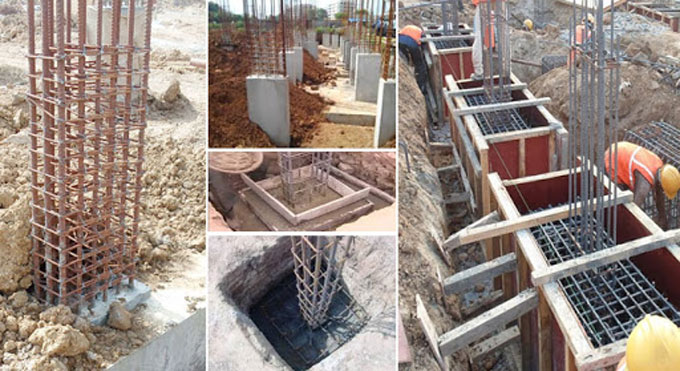# Reinforced Cement Concrete Column With FoundationReinforced cement concrete is also known as RCC. It is a versatile construction material. It is a combination of cement, sand, gravel, and water in particular quantities.

This type of concrete has a high strength and low tensile strength. If users add steel to the construction which has high tensile strength, the bond between the concrete and steels works very well. After combination of these two things, it is called reinforcement. After mixing the steel in the concrete the material is also known as reinforced cement concrete.

### Properties of RCC

1. Reinforced Cement Concrete must be capable of withstanding tensile, bending and shear forces.
2. Reinforced Cement Concrete also provides durability to the structure.
3. Any type of structure that built with RCC is able to support any load.
4. Reinforced Cement Concrete should develop hair cracks within the permissible limit.

A.) It provides stability and durability.
B.) It has high tensile strength.
C.) It is very affordable for the customers.
D.) It is fire resistant and prevent various environmental changes.

### Usage of Reinforced Cement Concrete

1. Reinforced Cement Concrete is used as element in beams, columns, footings, stairs, roofs, and slabs.
2. Reinforced Cement Concrete is also used for paving in roads, airports, and high traffic areas.
3. It is used to construct various structures like water tanks, bins, dams, bridges, retaining wall, underwater structures.

### Earthwork in excavation in foundation

1?2.00?2.00?0.83= 3.32CUM
(0.75M trapezoidal portion height+0.08M concrete)

Cement concrete 1:4:8 in base

1?2.00?2.00?0.08= 0.32CUM

R.C.C work 1:2:4: in footing excluding steel and it?s bending

Bottom square portion
1?2.00?2.00?0.25= 1.00CUM
Trapezoidal portion Vol
h/6(A1+A2+4Am)
0.5/6{(2?2+.35x.35+4[(2+.35)/2]x [(2+.35)/2]} =0.804CUM
Total: 1.00+0.804= 1.804CUM

R.C.C work 1:2:4: in column above Ground Level (G.L) excluding steel and it?s bending

Square portion below plinth level (P.L)
1x.35x.35?60 = 0.074 CUM
Circular portion above plinth level
1x(p/4?0.30?0.30) x3.30 = 0.233 CUM
Total: 0.074+0.233 = 0.307 CUM

Steel reinforcing bars including bending

(i) 12 mm dia bars @0.89kg in base footing
No. of bars = (200cm-8cm)/15cm+1= 14Nos.
(Bars are spaced at 15cm center to center and cover 8cm less, 4cm in one side + 1 bar is added)
L=200-2covers+2hooks = 200-2?4+2x9x1.2 = 213.6cm, say 2.14m
(cover=4cm, hook = 9xd, d is diameter of bar 12mm = 1.2cm)
Bars are placed two way so
2x14Nos.x2.14m (Bar length) = 2x14x2.14 = 53.33 Kg.

(ii) 16mm diameter dowel bars@ 1.58 kg
L= 0.45+0.75-2xcover+bend on the concrete
= 0.45+0.75-2x.04+0.15 (overlap 41cm+4cmcover=45cm)
= 1.27m, take 6 Nos. dowel bars 60? apart.
=6Nosx1.27?1.58 = 12.40kg

(iii) 16mm dia vertical bars @ 1.58kg in column
L = 3.30+0.6+0.10+0.10 =4.10m, take 6 Nos bars at 60? apart.
6Nosx4.1?1.58 = 38.87Kg

(iV) 10mm Dia bars @ 0.62kg in lateral ties
No.= 3.90/.15+1 = 27Nos.
L = px.22+18?1.0 (18xd) = .88m
27Nosx0.88?0.62 = 14.73kg.
Total weight: 53.33+12.04+38.87+14.73 = 118.97kg

### Abstract:

1. Earthwork in excavation in foundation: 3.32cum
2. Cement concrete 1:4:8 in base: 0.32cum
3. R.C.C work 1:2:4: in footing excluding 1.804cum

Steel and it?s bending.

4. R.C.C work 1:2:4: in column above Ground 0.307cum

Level (G.L) excluding steel and it?s bending

5. Steel reinforcing bars including bending- 118.97kg

Centering & shuttering required:

(a) Footing trapezoidal portion- 4Nos.x(2.00+0.35)/2xsloping height
=4?1.175?0.964
4.531 sqm

(b) Square portion above G.L up to plinth- =4Nos.x0.35?0.6
= 0.840 sqm

(c) Circular portion above plinth
=px0.3?3.3
=3.109sqm

Total= 4.531+0.84+3.109 = 8.48sqm

It is the most used building material for all types of structures and it is also used atomic plants for preventing danger from radiations.

### Wrap up